• coordinate
2021-11-15 10:32:11

#include
using namespace std;
class Coordinate {
public:
Coordinate()
{
times = 2;
cout << “Coordinate construction1 called!” << endl;
}
Coordinate(int times1)
{
times = times1;
cout << “Coordinate construction2 called!” << endl;
}
~Coordinate()
{
cout << “Coordinate destruction called!” << endl;
}
void inputCoord()
{
for (int i = 0; i < times; i++)
{
cout << “please input x:” << endl;
cin >> Coord[i];
cout << “please input y:” << endl;
cin >> Coord[i];
}
}
void ShowCoord()
{
cout << “the coord is:” << endl;
for (int i = 0; i < times; i++)
{
cout << “(” << Coord[i] << “,” << Coord[i] << “)” << endl;
}
}
void showavgcoord()
{
float avgx = 0;
float avgy = 0;
for (int i = 0; i < times; i++)
{
avgx - avgx + Coord[i];
avgy = avgy + Coord[i];
}
avgx = avgx / times;
avgy = avgy / times;
cout << “the avg coord is:” << endl;
cout << “(” << avgx << “,” << avgy << “)” << endl;
}
private:
float Coord;
int times;
};
int main()
{
Coordinate x;
x.inputCoord();
x.ShowCoord();
x.showavgcoord();
return 0;
}
1.结果显示，先执行的是构造函数，后执行析构函数。
2.当只执行新添加的代码，运行结果说明当次数输入5时，不再使用默认次数函数，而是使用第二个设置次数函数。
当一起执行原代码加新添加的代码，结果显示，先调用两次构造函数的输入，最后连续调用两次析构函数。

更多相关内容
• This is a repo that doing some Geomatics coordinate transform by C#, Including: Four-parameter coordinate transformation Three-parameter coordinate transformation Seven-parameter coordinate ...C#
• matlab开发-coordinateTransformation。多普勒效应测量装置的坐标变换。
• 然后使用require('tg-coordinate-picker')在 javascript 文件中require('tg-coordinate-picker')插件。 用法 创建一个具有合适宽度和高度的 div 来保存谷歌地图。 如果 div 有一个类coordinate-picker ，那么只需在...JavaScript
• 2017-A generic coordinate descent framework for learning from implicit feadback-WWWJavaScript
• China Geodetic Coordinate System 2000.prj .........................
• This Letter proposes a coordinate difference homogenization matching method to solve motion influence in three-dimensional (3D) range-intensity correlation laser imaging. Firstly, features and feature...
• 此Python 3.1工具可操纵CNC GCODE的坐标系进行加工或雕刻。 它可以翻转X，Y或Z坐标，镜像X，Y或Z坐标，翻转或镜像两个XY坐标，或将Z动作插入没有它们的GCODE文件中。开源软件
• 套索坐标下降 在这些示例中，使用循环和随机坐标下降算法解决了具有L1正则化的LASSO问题。 该算法的一般形式为： 例子： 此存储库中有三个示例： 使用真实数据集的演示 使用模拟数据集的演示 ...Python
• 代码，下载后即可直接在Qt Creator中打开、编译运行。 动态折线图可缩放可拖拽，静态折线图实时性更高。 对应博文地址：https://blog.csdn.net/qq_37385181/article/details/83055915
• 将WGS-84(国际标准)转为GCJ-02(火星坐标)，将GCJ-02(火星坐标)转为百度坐标，将百度坐标转为GCJ-02(火星坐标)，将GCJ-02(火星坐标)转为WGS-84
• Blockwise Coordinate Descent Schemes for Sparse Representation研究论文
• 自己做的一些关于绝对节点坐标法的整理，希望对大家有用，欢迎交流讨论
• 主要完成常见坐标系的转换，包括： WGS84(国际通用坐标系)<--->GCJ02(中国火星坐标系，高德地图坐标系)<--->BD09(百度地图坐标系)matlab
• 协调客户端（浏览器）JS 应用程序的路由器。... 获得 repo 的副本后，在开始工作之前运行以下命令以确保一切都处于工作状态： make installmake test在对 fork/branch 进行更改之前，请运行以下命令以确保没有损坏： ...JavaScript
• NULL 博文链接：https://san-yun.iteye.com/blog/288382
• android-coordinate-layout 坐标布局是超级强大的Framelayout。 坐标布局可让视图根据其他视图的布局/位置的变化做出响应。 小吃店和Fab。 IN Snackbar坐标布局视图以VIew的形式传递。 这样，坐标布局就可以了解...Java
• 坐标转换器此可执行文件用于将坐标从 UTM 格式批量转换为 Lat Lon 并将 Lat Lon 转换为 UTM 格式。 输入文件应包含格式如下例所示的坐标： 经纬度到 UTM 转换的输入文件格式： Point Id Latitude Longitude Altitude...
• The specific process is: from the world coordinate system to the camera coordinate system, then from the camera coordinate system to the image coordinate system, and finally from the image coordinate...

The process of three-dimensional reconstruction using image sequences is equivalent to a process of restoring two-dimensional images composed of many pixels to three-dimensional space. By understanding the entire projection process, it is easy to understand how to use images for 3D reconstruction and what are the key steps in 3D reconstruction. This section mainly describes the projection process of a monocular camera.

# A. Pinhole imaging model and coordinate system

The process of taking an image by a camera can be simplified into a form of pinhole imaging, and the mathematical expression of the camera model can be easily obtained by using this form. Through the imaging method of the camera and its mathematical expression, the mapping relationship between the three-dimensional scene and each pixel in the image can be seen. Through this mapping relationship, the pixels in the image can be restored to three-dimensional space. If all the pixels of a series of images are restored to the entire three-dimensional space, the surface of the entire scene can be restored. The camera's pinhole imaging model structure is shown in Figure 2-1. In order to simplify it, the imaging plane is placed in front of the pinhole, and the captured image should also be upright.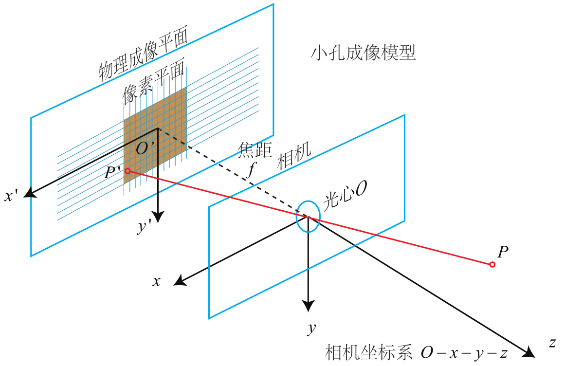Fig. 1 The image of the pinhole imaging model

In the pinhole imaging model, the whole process of projecting the scene from the three-dimensional space to the picture can be roughly decomposed into three steps and four coordinate systems. The specific process is: from the world coordinate system to the camera coordinate system, then from the camera coordinate system to the image coordinate system, and finally from the image coordinate system to the pixel coordinate system. The process of projecting the scene in front of the camera lens to a 3D image can be regarded as a transformation process of the 3D scene from the world coordinate system to the pixel coordinate system. The four coordinate systems are defined as follows.

The world coordinate system is an objective absolute existence. The world coordinate system needs to be predetermined, specifying its origin and orientation. In the defined world coordinate system, any object can be placed. In 3D reconstruction or computer vision, the camera coordinate system of the first frame image opened by the photographic camera is usually predefined as the world coordinate system. Then calculate the next movement path and pose of the camera according to the defined world coordinate system. Coordinates in the world coordinate system are usually represented by (Xw, Yw, Zw).

The current common method of defining the coordinate system of a camera is to use the optical center or principal point of a camera as the origin of the coordinate system. The X-axis and Y-axis are parallel to the horizontal and vertical axes of the image, respectively, when the picture is captured. The Z axis is the direction in which the camera's focal length points. Coordinates in the camera coordinate system are usually represented by the notation (Xc, Yc, Zc).

The image coordinate system is defined according to the image, with the center of the image as the origin, and the X and Y axes are in the same direction as the X and Y axes of the camera coordinate system. An image coordinate system is a coordinate system expressed in physical units such as meters and centimeters. The image coordinate system has only two dimensions and no Z axis. Coordinates in the image coordinate system are usually represented by the notation (x, y).

A pixel coordinate system is a coordinate system in pixels. The pixel coordinate system is also a two-dimensional coordinate system, and the directions of its X-axis and Y-axis are consistent with the image coordinate system, and the origin of the coordinate system is located in the upper left corner of the two-dimensional image. The general representation of a pixel is a square or a rectangle, and the information stored in each pixel is the intensity or gray value of the pixel.

# B. Coordinate system transformation

In subsection A, each coordinate system and the representation of the point in the coordinate system have been defined. In this subsection, the entire process of pinhole imaging is deduced in the form of a mathematical model. The whole process is divided into three steps. The process of changing from the world coordinate system to the camera coordinate system involves the external parameters of the camera. The process of converting from the camera coordinate system to the image coordinate system and then to the pixel coordinate system involves the camera's internal parameters. usage of. This section derives the camera's projection process in the form of camera intrinsic and extrinsic parameters.

## (1) Camera external parameters

Conversion of the world coordinate system (Xw, Yw, Zw) and the camera coordinate system (Xc, Yc, Zc): The camera coordinate system is an unstable coordinate system, because the position of the origin and each coordinate axis will change as the camera moves direction. In the process of 3D reconstruction or camera positioning, a stable and unchanging world coordinate system is required. In the world coordinate system, all coordinates can be unified.

Suppose point P is a point in three-dimensional space, its position in the camera coordinate system is Pc , and its position in the world coordinate system is Pw. Pw and Pc can be converted to each other by a transformation matrix, which can be subdivided into a rotation matrix (R) and a translation matrix (t). Its mathematical expression is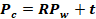​                                                                                                    (1)

where R has three degrees of freedom and is a 3×3 matrix representing the rotation of the camera in the world coordinate system. t​ Represents the origin of the camera relative to the world coordinate system or the origin of the coordinate system at the beginning of the camera, which is a 3×1 matrix. Expanding the above formula into a specific form is: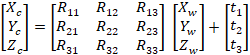​                                                                               (2)

Its homogeneous coordinate form can be expressed as: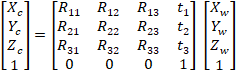​                                                                              (3)

This can be simplified to: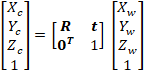​                                                                                         (4)

where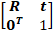​ is called the camera's extrinsic parameter matrix, denoted by T.

## (2) Camera internal parameters

Conversion of camera coordinate system (Xc, Yc, Zc) and image coordinate system (x, y): The camera coordinate system is a three-dimensional coordinate system, the image coordinate system is a two-dimensional coordinate system, and there is a dimensionality reduction process from three-dimensional to two-dimensional , mainly because the dimension of depth is lost, and the coordinate vector is converted from three-dimensional to two-dimensional.

As shown in Figure 2, there is any point ​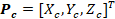in the camera coordinate system, which is arbitrary, and the coordinate point ​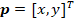on the image corresponds to this three-dimensional point. In Section A, we know that the X​ and Y​ axes of the two coordinate systems are the same, so the image point p​ can add a dimension f​ to form the coordinates of the camera coordinate system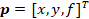, where f​ is the focal length of the camera.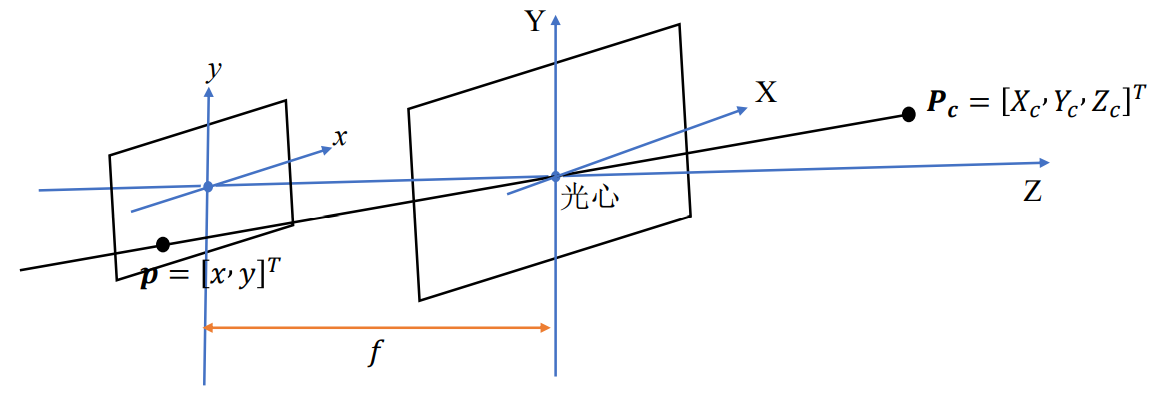Figure 2 Schematic diagram of the conversion between the camera coordinate system and the image coordinate system

From the similarity relationship of the triangles, the following formula can be obtained: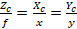​                                                                                                  (5)

After arranging the above formula, we can get: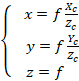​                                                                                                (6)

Convert it to a homogeneous coordinate system as: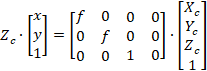​                                                                               (7)

So far, the transformation mathematical model of camera coordinate system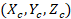and image coordinate system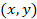has been deduced.

​Conversion of image coordinate systemand pixel coordinate system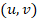: The core difference between these two coordinate systems is that their units are inconsistent. Both the image coordinate system and the pixel coordinate system are two-dimensional coordinate systems, and their representation units are respectively physical units represented by centimeters, meters, etc., and pixel units based on pixels. As shown in Figure 3, according to the definition in Section A, the center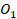of the image is the coordinate origin of the image coordinate system, and the upper left corner of the image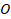is the coordinate origin of the pixel coordinate system.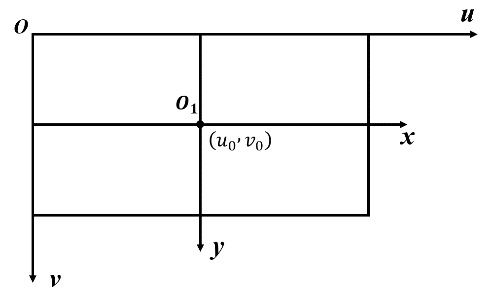Figure 3 Schematic diagram of conversion between image coordinate system and pixel coordinate system

​Assume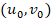​ is the coordinates of the pixel coordinate system, representing the center of the entire image. The representation of a pixel is usually a rectangle or a rectangle, so the length and width of a pixel are represented as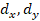respectively. Then according to the above-defined symbols, any pointin the image coordinate system can be in a one-to-one correspondence with any pointin the pixel coordinate system. Its mathematical conversion form is: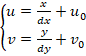​                                                                                                  (8)

This form of addition is not conducive to the calculation of the computer, so similar to the above calculation using the homogeneous coordinate system, the homogeneous coordinate does not change the degree of freedom of the vector while increasing the dimension of the vector. The above formula can be converted into: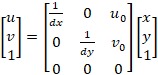​                                                                                          (9)​
Equation (9) converts the addition operation in the vector into a multiplication operation, which is convenient for the operation of the computer. The mathematical model of the conversion between the image coordinate systemand the pixel coordinate systemis obtained by formula (2-9).

Through equations (7) and (9), the entire projection process of a point in a three-dimensional space from the camera coordinate system to the pixel coordinate system can be obtained, and its mathematical form is: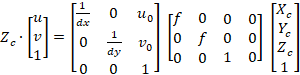​                                                                        (10)

By integrating the intermediate transformation matrix, we can get: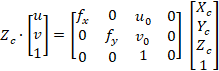​                                                                              (11)

where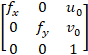​ It is called the camera's internal parameter matrix and is represented by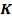.

​Through the deductive derivation of the above formula, you can get a camera's internal parameter matrix, the camera's internal parameter matrix ​has 4 unknown data, and these four data are related to the structure of the camera. ​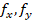is a short form of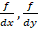​, ​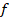is the focal length of the camera, and ​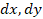is the length and width of the unit pixel.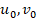​ is the unknown coordinate in the middle of the image in the pixel coordinate system. Usually the internal parameters of the camera have been calibrated when the camera leaves the factory and are known quantities.

## (3) Combination of internal and external parameters

Through the internal and external parameters of the camera, all the transformations between the four coordinate systems in the camera model can be linked. The mathematical form is: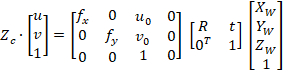​                                                                   (12)

From the formula (12), it can be known that the three-dimensional spatial point can be projected into the pixel space of the image through the internal and external parameters of the camera. The internal parameters of the camera are generally known, and the scene in the image can be restored to the three-dimensional space by knowing the external parameters of the camera and the depth of the pixel in the image coordinate system. The process of solving the camera extrinsic parameters is actually a process of positioning the camera, which is usually called visual odometry. Solving for image depth is called depth estimation.

展开全文计算机视觉
•gis
• matlab中平行坐标图的实现方法，可以在此基础上进行变通，从大神那学来，仅供学习交流。
• C坐标转换 C＃坐标转换程序4参数7参数在进行坐标转换时，经常涉及到使用C＃进行4参数和7参数计算的程序，计算方便
• Kinect 2 坐标映射该项目使用 SDK 2.0 版演示 Kinect for Windows 坐标映射。 了解如何在屏幕上绘制关节并将它们与颜色或深度框架完美对齐。教程阅读完整教程以了解 Kinect 坐标映射：[ ] ( )例子 foreach (Joint ...C#
• 坐标转换源码,测量中常用的转化坐标，三参数、七参数求解
• 抗差估计在坐标转换中的应用，张鹤鸣，王中元，粗差会对数据处理造成很大的影响，抗差估计提供了消除和削弱观测误差中粗差对参数估计的干扰和影响，求解最佳估值的方法。本文运
• gm中关于国家2000坐标系cgcs2000的设置方法，希望对您有用cgcs2000...